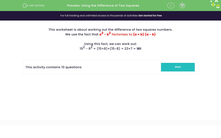# Using the Difference of Two Squares

In this worksheet, students practise using the difference of two squares.Key stage:  KS 3

Curriculum topic:   Number

Curriculum subtopic:   Understand Integer Powers/Real Roots

Difficulty level:#### Worksheet Overview

This worksheet is about working out the difference of two squares numbers.

We use the fact that a2 - b2 factorises to (a + b) (a - b)

Using this fact, we can work out:

152 - 82 = (15+8)×(15-8) = 23×7 = 161

### What is EdPlace?

We're your National Curriculum aligned online education content provider helping each child succeed in English, maths and science from year 1 to GCSE. With an EdPlace account you’ll be able to track and measure progress, helping each child achieve their best. We build confidence and attainment by personalising each child’s learning at a level that suits them.

Get started